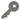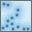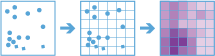# Calculate DensityAvailable in big data analytics.

The Calculate Density toolcalculates a magnitude-per-unit area from point features that fall within a neighborhood around each cell.

## Workflow diagram## Examples

• Bird counts can be used to calculate species densities. The densities can be compared to land-cover data to determine which habitats each species prefers.
• The density of GPS measurements of flight paths can be calculated to determine which areas of airspace have high volumes of traffic.

## Usage notes

• The Calculate Density tool requires a single input of point features.
• Input points are aggregated into bins for analysis. You must specify the Bin Size into which to aggregate data.
• As shown in the diagram below, if you are aggregating into hexagons, the bin size d is the height of each hexagon, and the width of the resulting hexagon will be two times the height divided by the square root of three. If you are aggregating into squares, the bin size d is the height of the square, which is equal to the width.

• You must specify a Radius value that is greater than the bin size. The radius is used to find input features within the same neighborhood as the feature (bin) of interest.
• There are two options to calculate the density Weight value: The Uniform option sums all the values within the neighborhood and divides them by the area. The Kernel option weights values in the neighborhood by distance from the feature of interest and applies a kernel function to fit a smooth tapered surface to each point.
• Density can be calculated using one or more count fields. A count field is a numerical field that specifies the number of incidents at each location. You can use a count field for features such as cities or highways when calculating the density of population or lanes of traffic, respectively. If you specify a count field, the density will be calculated for the count field in addition to the density of points.
• You can use the Calculate Density tool to analyze using time stepping. Each time slice is analyzed independent of features outside of the time step. To use time stepping, the input data must be time enabled and represent an instant in time. When time stepping is applied, output features will be time intervals represented by the StartTime and EndTime fields.
• Only areas within the neighborhood of a bin containing points will be returned.
• The Calculate Density tool requires the data be in a projected coordinate system. If the data is in a geographic coordinate system, use the Project tool first to project the data to a projected coordinate system.

## Parameters

ParameterDescriptionData type

Input Layer

The point features for which density will be calculated.

Features

Weight

The weighting applied to the density function.

• Uniform—A magnitude-per-area calculation in which each bin is equally weighted. This is the default.
• Kernel—A magnitude-per-area calculation with a smoothing algorithm applied (kernel) that weights bins closer to the points more heavily.

String

Bin Type

The bin shape used to create the regular bins. The options are Square or Hexagon.

String

Bin Size

The distance interval that represents the bin size into which the input points will be analyzed.

String

The search radius applied to density calculations.

String

Output Units

The area units of the output density values.

String

Population Field(s) (optional)

One or more fields denoting population values for each feature. The population field is the count or quantity to be spread across the landscape to create a continuous surface.

Values in the population field must be numeric. By default, the density of the count of input points will be calculated.

String

Interval (optional)

A value that specifies the duration of the time step. This option is only available if the input points are time enabled and represent an instant in time.

Time stepping can only be applied if time is enabled on the input. For more information on how time stepping works, see How time stepping works.

String

Repeat (optional)

Specifies how often the time-step interval occurs. This parameter is only available if the input points are time enabled and represent an instant in time.

String

Alignment (optional)

A date specifying the reference time with which to align the time steps. The default is January 1, 1970, at 12:00 a.m. This option is only available if the input points are time enabled and represent an instant in time.

Int64

## Output layer

The output layer will contain the following fields in place of the original fields:

Field nameDescriptionField type

density_COUNT

The magnitude-per-unit area from point features that fall within a neighborhood around each cell.

If fields are specified in the Population Field(s) parameter, this field will not be generated.

Float64

density_SUM_<countField(s)Name>

The magnitude-per-unit area from point features that fall within a neighborhood around each cell for the count field.

This field will only be generated if fields are specified in the Population Field(s) parameter.

Float64

## Considerations and limitations

Density can only be calculated for point features.